• anaconda matplotlib 添加字体
千次阅读
2019-11-06 15:49:48

## 1. 下载字体

https://www.fontpalace.com/font-download/SimHei/


## 2. 安装字体

### 2.2 如果其他可以把自己拷贝到指定目录

• 先找到目录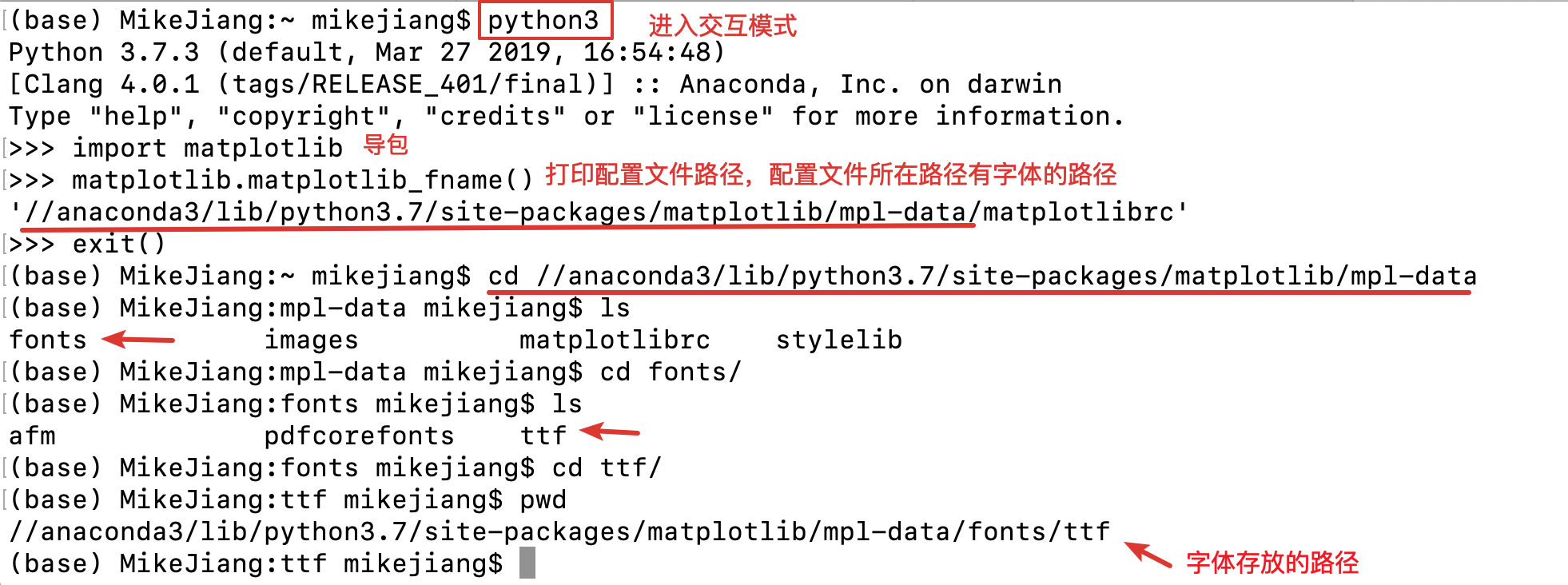import matplotlib
matplotlib.matplotlib_fname()

• 把字体拷贝到指定目录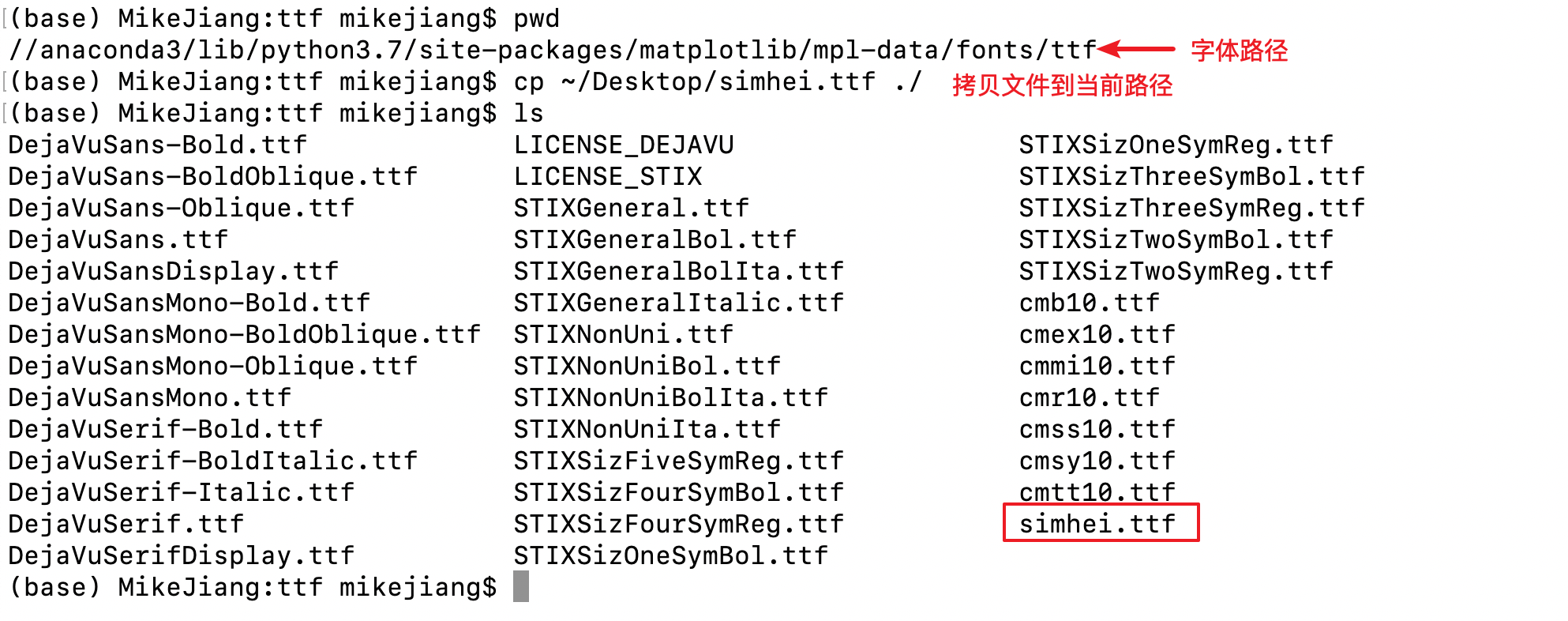## 3. matplotlibrc配置文件修改

### 3.1 查找配置文件路径

import matplotlib
matplotlib.matplotlib_fname()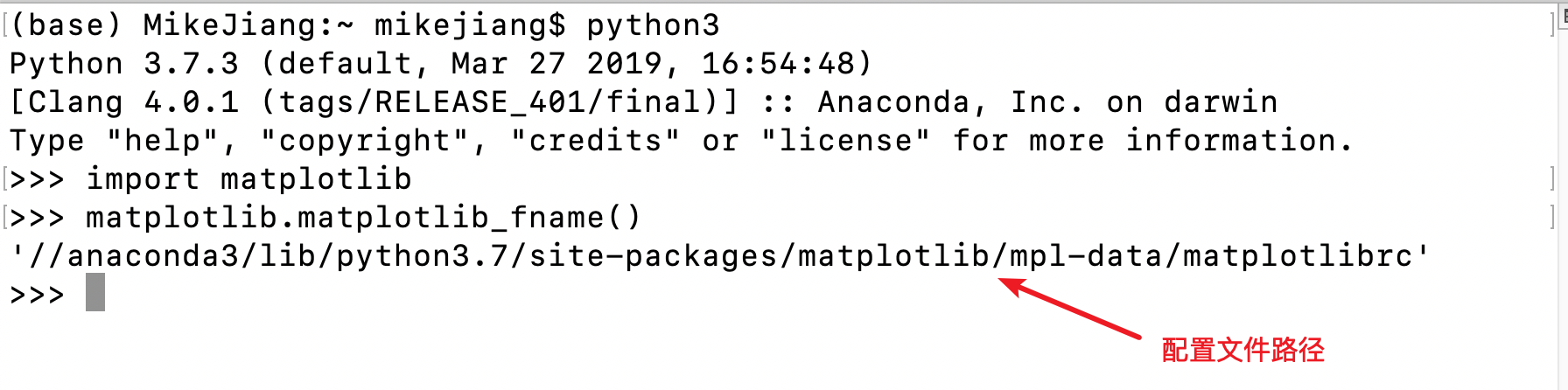### 3.2 修改配置文件

在合适位置添加如下内容：

font.family         : sans-serif
font.sans-serif     : SimHei
axes.unicode_minus  : False


## 4. 重新加载字体

from matplotlib.font_manager import _rebuild
_rebuild()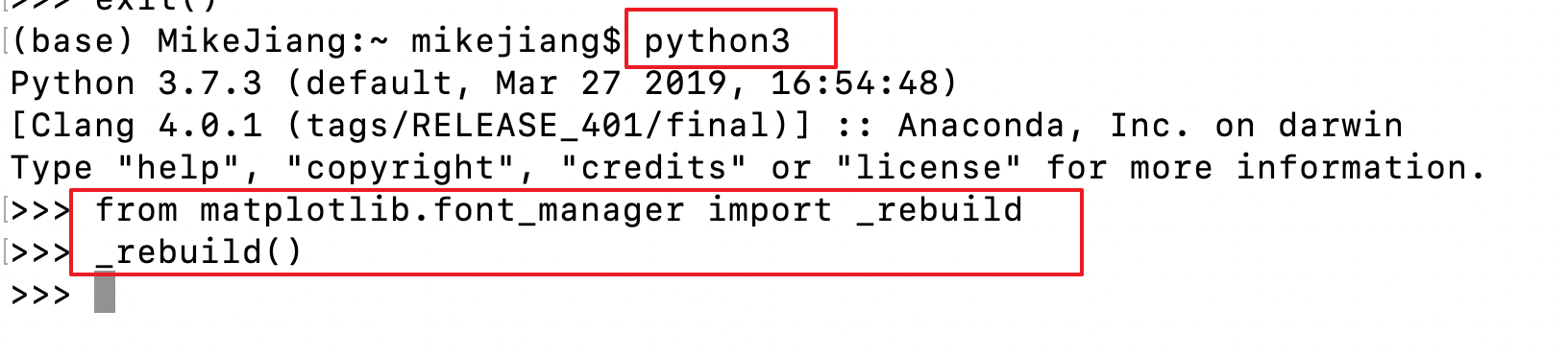## 5. 参考资料matplotlib anaconda
更多相关内容
• 提供三种解决方案，其中一种可以直接设置matplotlib默认字体，一劳永逸

# 问题描述

import numpy as np
from matplotlib import pyplot as plt

x = np.arange(1, 11)
y = 5 - x
plt.title('标题 - 中文')
plt.xlabel('X轴')
plt.ylabel('Y轴')
plt.plot(x, y)
plt.savefig('result.jpg', bbox_inches='tight')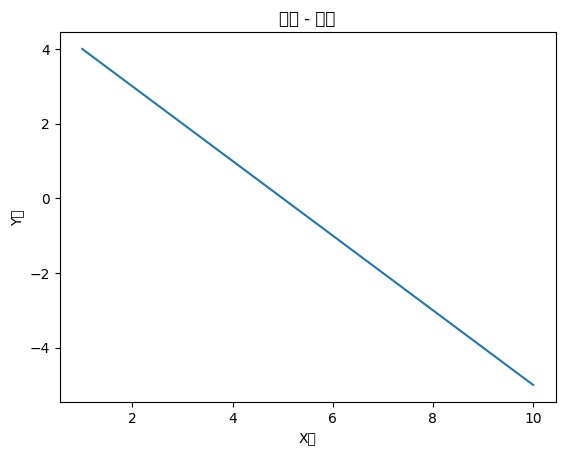Windows下添加两行代码可轻松解决

plt.rcParams['font.sans-serif'] = ['SimHei']  # 无衬线字为微软雅黑
plt.rcParams['axes.unicode_minus'] = False  # 正确显示负号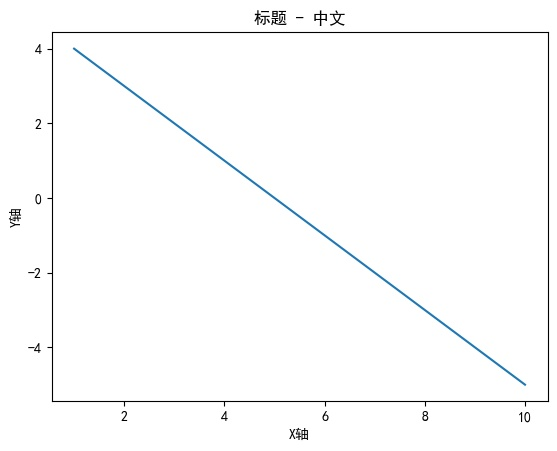Linux下如何解决呢？

# 解决方案

## 1. 指定字体

下载任意一个思源黑体，如 SourceHanSansSC-Normal.otf

import numpy as np
from matplotlib import pyplot as plt
from matplotlib.font_manager import FontProperties

fontproperties = FontProperties(fname='SourceHanSansSC-Normal.otf')
x = np.arange(1, 11)
y = 5 - x
plt.title('标题 - 中文', fontproperties=fontproperties)
plt.xlabel('X轴', fontproperties=fontproperties)
plt.ylabel('Y轴', fontproperties=fontproperties)
plt.plot(x, y)
plt.savefig('result.jpg', bbox_inches='tight')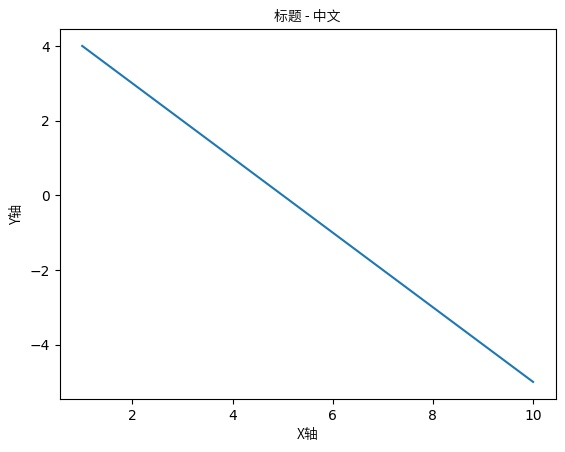## 2. 使用系统字体

查看可用的系统中文字体

import subprocess
from matplotlib.font_manager import FontManager

matplotlib_fonts = {i.name for i in FontManager().ttflist}

output = subprocess.check_output('fc-list :lang=zh -f "%{family}\n"', shell=True)
system_fonts = {i.split(',', 1) for i in output.decode().split('\n')}

fonts = matplotlib_fonts & system_fonts
for i in fonts:
print(i)


结果

Noto Sans CJK JP


调用

import numpy as np
from matplotlib import pyplot as plt

plt.rcParams['font.family'] = ['Noto Sans CJK JP']
x = np.arange(1, 11)
y = 5 - x
plt.title('标题 - 中文')
plt.xlabel('X轴')
plt.ylabel('Y轴')
plt.plot(x, y)
plt.savefig('result.jpg', bbox_inches='tight')


效果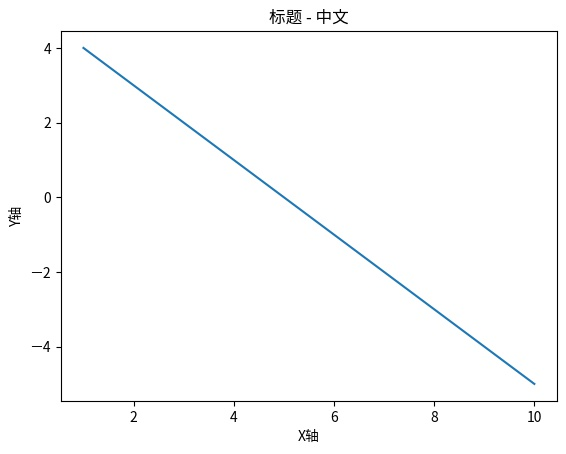## 3. Anaconda设置matplotlib中文字体（一劳永逸）

复制 C:\Windows\Fontssimhei.ttf 即黑体

放到 /home/xercis/anaconda3/envs/py38/lib/python3.8/site-packages/matplotlib/mpl-data/fonts/ttf/

修改 /home/xercis/anaconda3/envs/py38/lib/python3.8/site-packages/matplotlib/mpl-data/matplotlibrc

搜索 font.sans-serif

改为

font.family: sans-serif
font.sans-serif: SimHei, DejaVu Sans, Bitstream Vera Sans, Computer Modern Sans Serif, Lucida Grande, Verdana, Geneva, Lucid, Arial, Helvetica, Avant Garde, sans-serif


搜索 axes.unicode_minus，将True改为False，解决负号 - 显示为方块的问题

删除 /home/xercis/.cache/matplotlib/

运行

import numpy as np
from matplotlib import pyplot as plt

x = np.arange(1, 11)
y = 5 - x
plt.title('标题 - 中文')
plt.xlabel('X轴')
plt.ylabel('Y轴')
plt.plot(x, y)
plt.savefig('result.jpg', bbox_inches='tight')


效果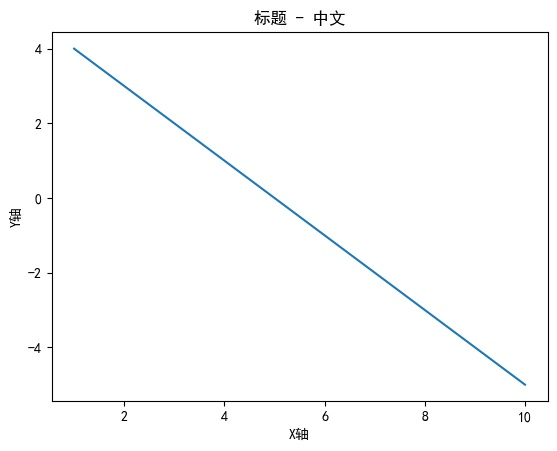# 参考文献

展开全文python matplotlib
• 基于Anacondamatplotlib学习matplotlib安装入门小案例 matplotlib安装 第一步：找到开始桌面下的anaconda下面的anaconda prompt！ 第二步：输入如下命令。 conda install matplotlib 入门小案例 案例一：大家...

## matplotlib安装

第一步：找到开始桌面下的anaconda下面的anaconda prompt！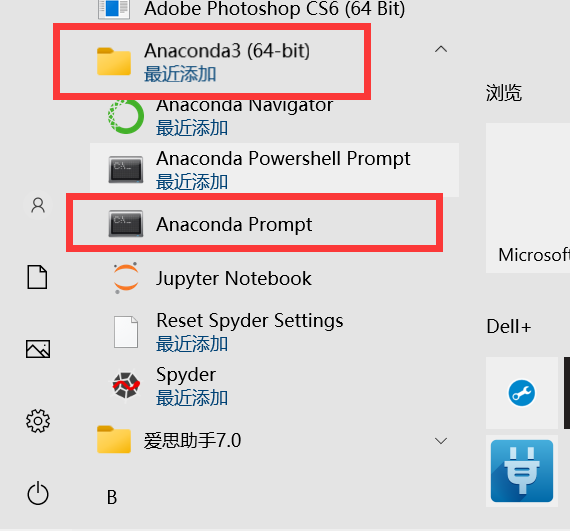第二步：输入如下命令。

conda install matplotlib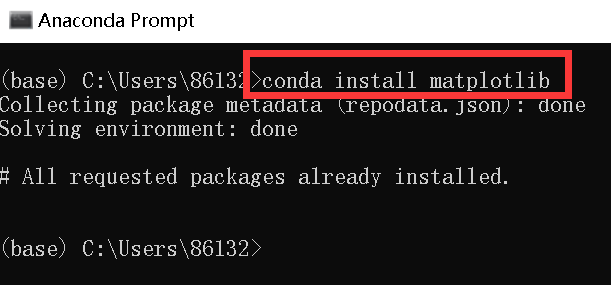## 入门小案例

案例一：大家结合注释好好理解步骤！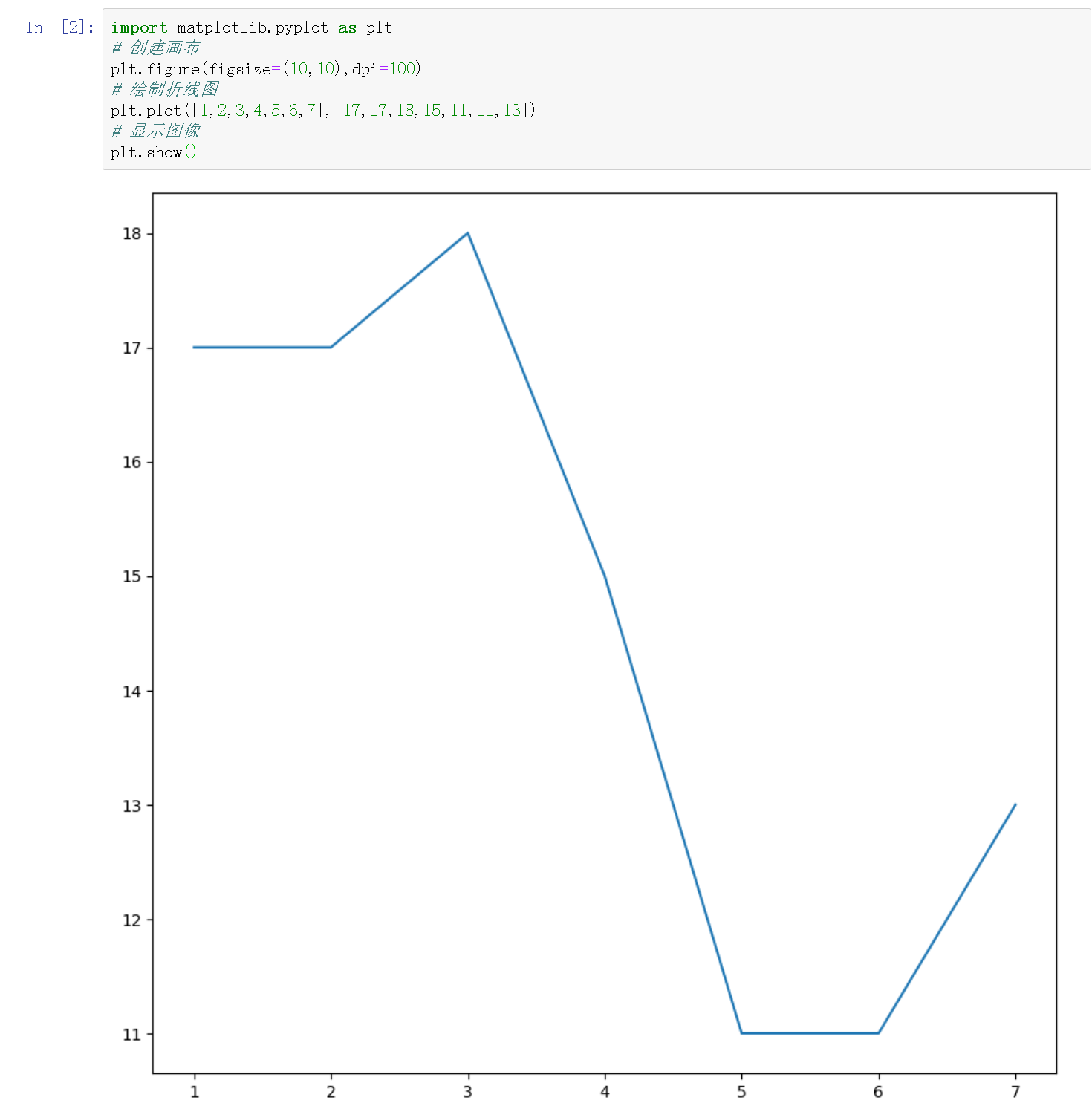对于不懂的函数，也可以先输入函数后，shift和左括号，然后弹出来提示后，再点击+号来看奥！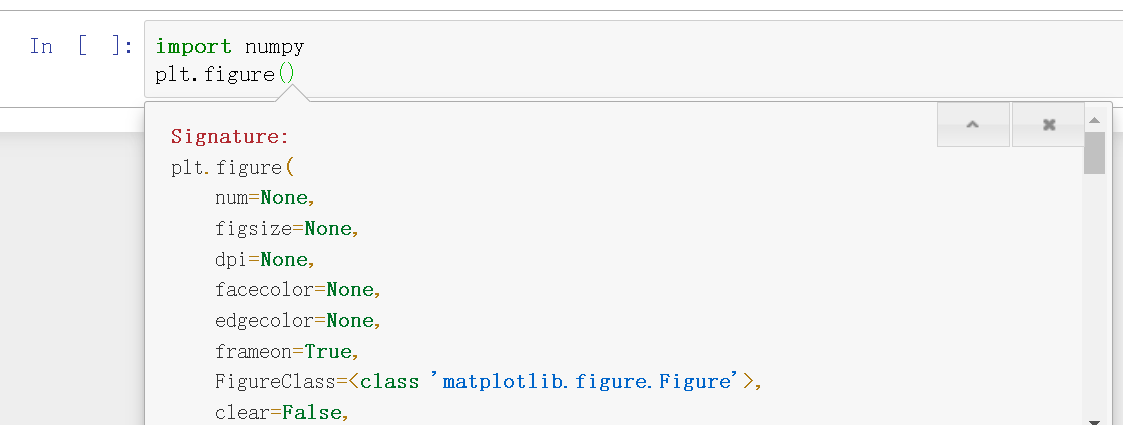案例二：plot函数的参数是x和y，故可以提前准备好一些数据，这里的数据准备可以根据numpy中的函数来实现。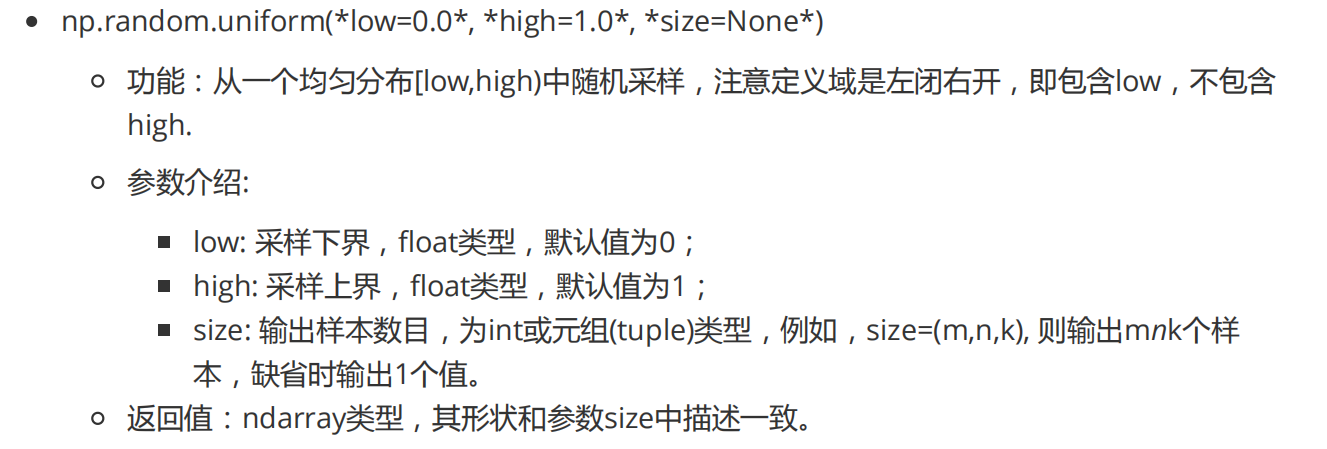import numpy as np
x=np.arange(1,51)
y=np.random.uniform(10,20,(x.size,))
plt.figure(figsize=(20,8),dpi=80)
plt.plot(x,y)
plt.show()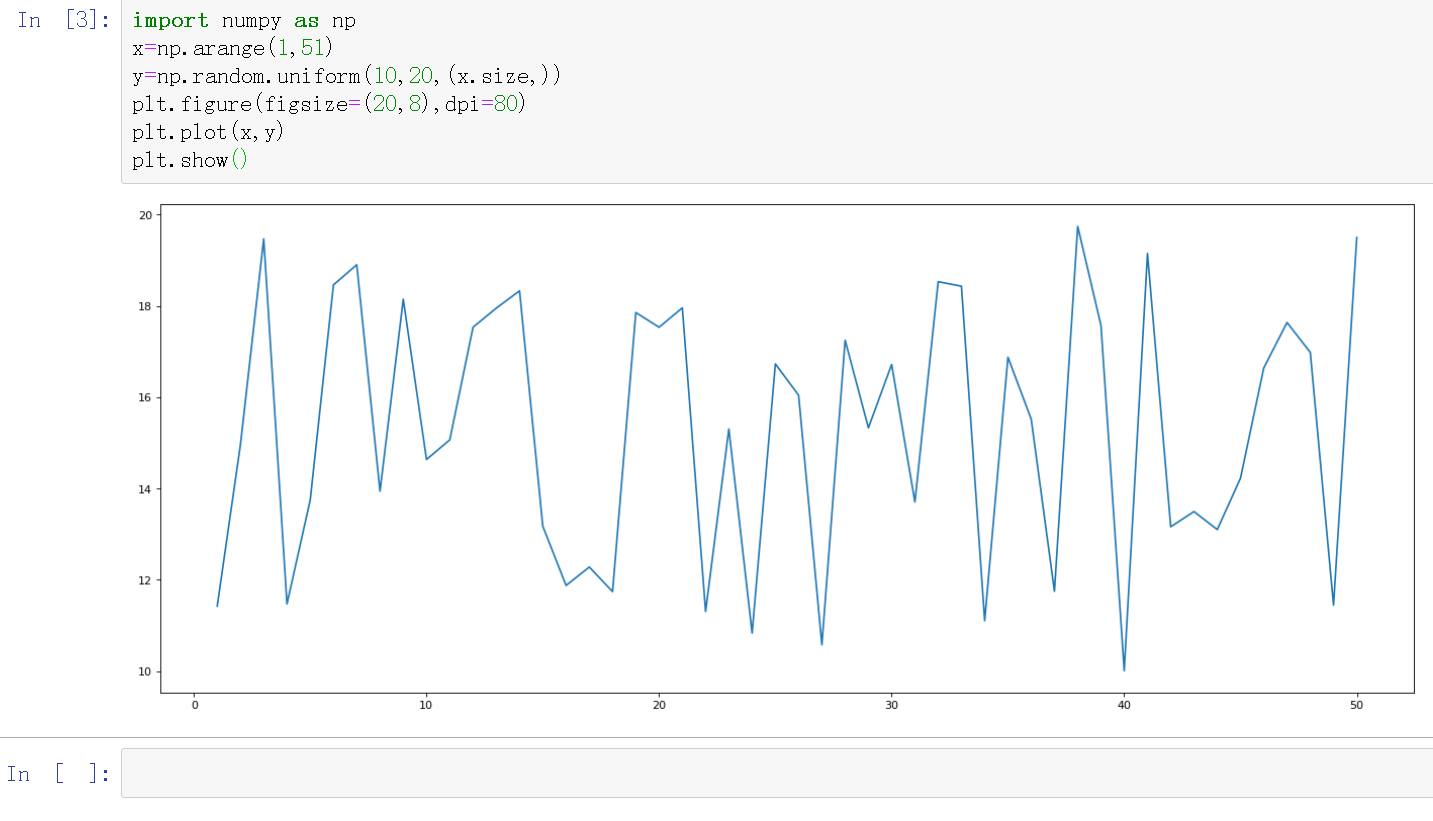案例三：如何在绘图基础上，给其增加刻度值呢！

from pylab import mpl
# 设置显示中文字体
mpl.rcParams["font.sans-serif"]=["SimHei"]

x=np.arange(60)
y=np.random.uniform(10,20,(x.size,))
plt.figure(figsize=(20,8),dpi=80)
plt.plot(x,y)

# 添加x和y刻度 放在plot和show之间
x_=x[::5]
y_=[i for i in range(21)]

x_t =[ '11点{}分'.format(i) for i in x_]
y_t=['{}℃'.format(i) for i in y_]
# 绘制刻度值
plt.xticks(x_,x_t) # x_必须是数字 但是可以使用中文x_t来替换x_
plt.yticks(y_,y_t)

plt.show()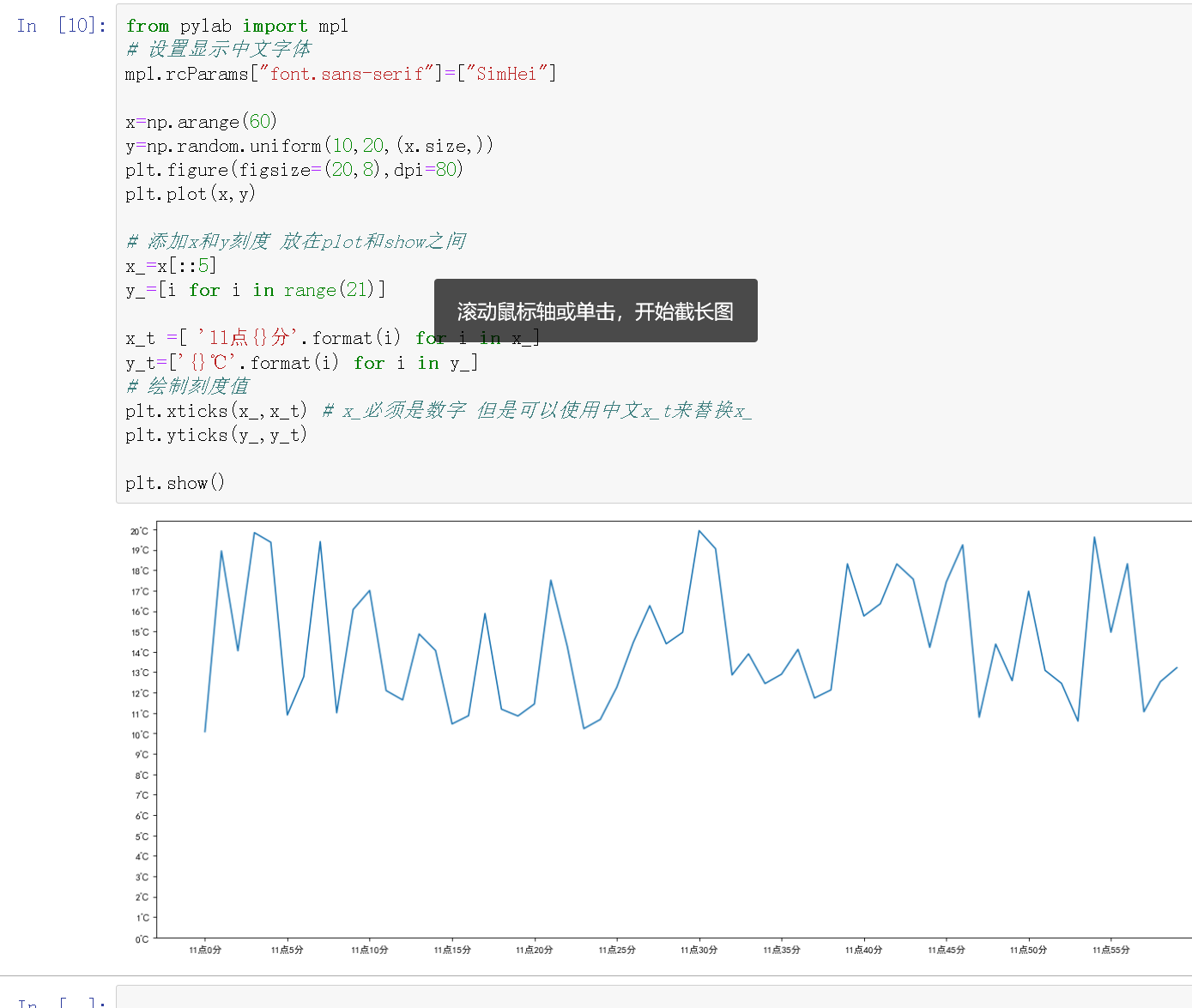如果我们觉得下面太空了，或者太密了，可以做如下调整！

from pylab import mpl
# 设置显示中文字体
mpl.rcParams["font.sans-serif"]=["SimHei"]

x=np.arange(60)
y=np.random.uniform(10,20,(x.size,))
plt.figure(figsize=(20,8),dpi=80)
plt.plot(x,y)

# 添加x和y刻度 放在plot和show之间
x_=x[::2]
y_=[i for i in range(10,21)]

x_t =[ '11点{}分'.format(i) for i in x_]
y_t=['{}℃'.format(i) for i in y_]
# 绘制刻度值
plt.xticks(x_,x_t,rotation=45) # x_必须是数字 但是可以使用中文x_t来替换x_
plt.yticks(y_,y_t)

plt.show()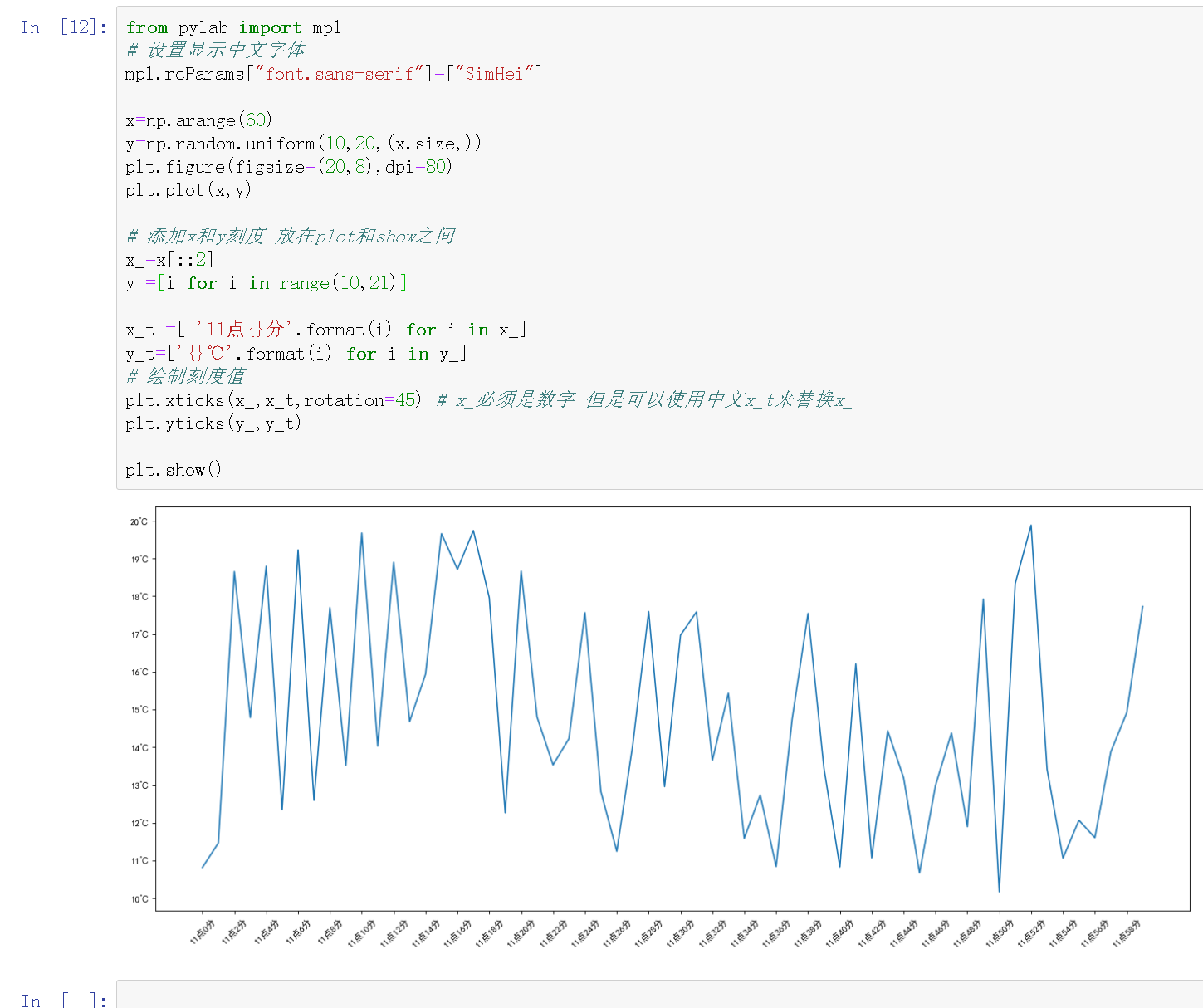这样会不会更好看呢？

from pylab import mpl
# 设置显示中文字体
mpl.rcParams["font.sans-serif"]=["SimHei"]

x=np.arange(60)
y=np.random.uniform(10,20,(x.size,))
plt.figure(figsize=(20,8),dpi=80)
plt.plot(x,y)

# 添加x和y刻度 放在plot和show之间
x_=x[::2]
y_=[i for i in range(10,21)]

x_t =[ '11点{}分'.format(i) for i in x_]
y_t=['{}℃'.format(i) for i in y_]
# 绘制刻度值
plt.xticks(x_,x_t,rotation=45) # x_必须是数字 但是可以使用中文x_t来替换x_
plt.yticks(y_,y_t)

# 设置坐标轴名称
plt.xlabel("时间",fontsize=16)
plt.ylabel("温度",fontsize=16)

# 设置标题
plt.title("11点到12点内各时段温度变化情况",fontsize=20)

# 设置网格线 放在show前面
plt.grid(True,linestyle='--')

plt.show()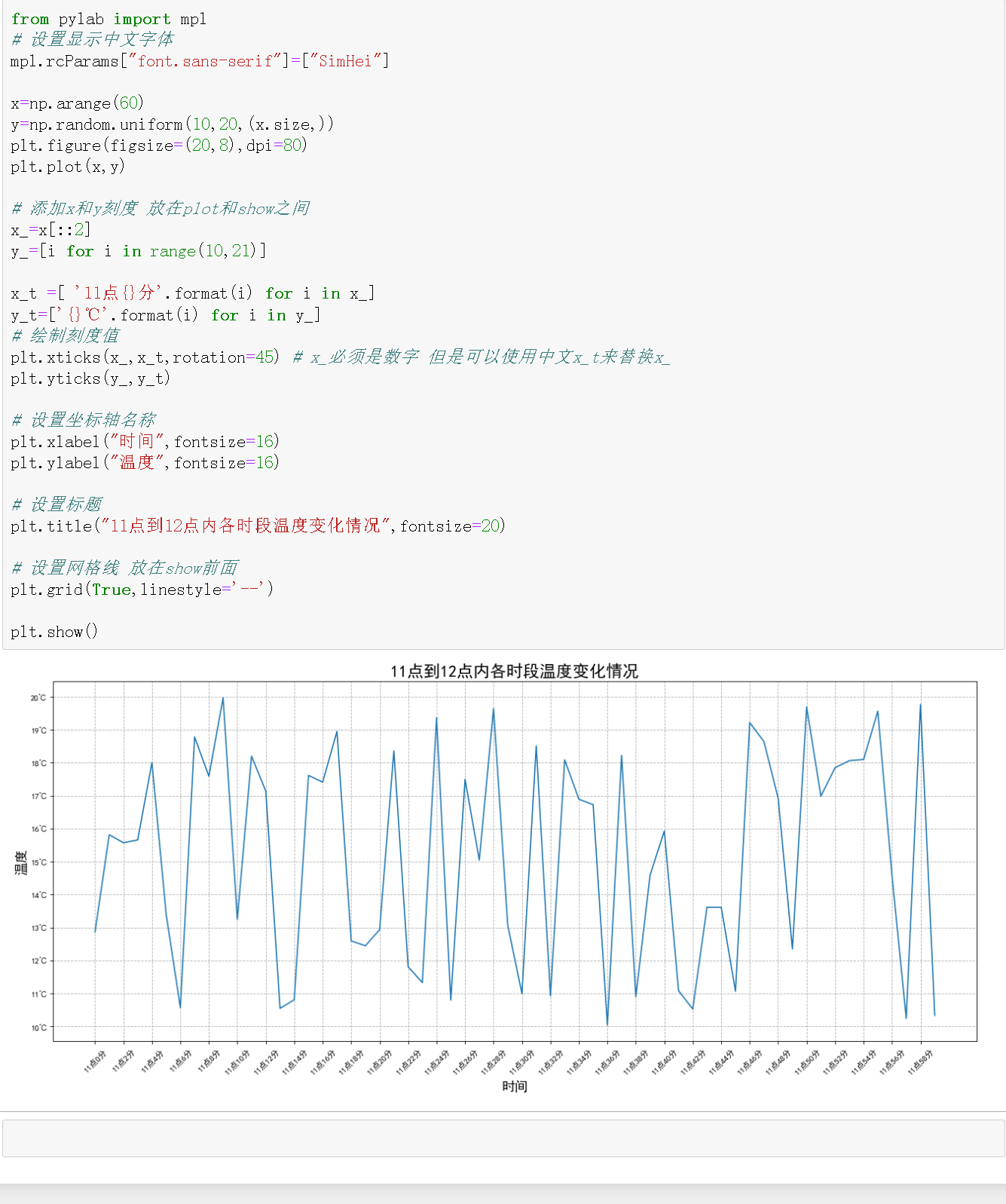当绘制多个图时，对应的扩充！

from pylab import mpl
# 设置显示中文字体
mpl.rcParams["font.sans-serif"]=["SimHei"]

x=np.arange(60)
y=np.random.uniform(10,20,(x.size,))
y_wu=np.random.uniform(10,20,(x.size,))

plt.figure(figsize=(20,8),dpi=80)

# 当绘制多个图时可添加图例 使用label标签配合legend
plt.plot(x,y,label='北京')
plt.plot(x,y_wu,label='武汉')

# 添加x和y刻度 放在plot和show之间
x_=x[::2]
y_=[i for i in range(10,21)]

x_t =[ '11点{}分'.format(i) for i in x_]
y_t=['{}℃'.format(i) for i in y_]
# 绘制刻度值
plt.xticks(x_,x_t,rotation=45) # x_必须是数字 但是可以使用中文x_t来替换x_
plt.yticks(y_,y_t)

# 设置坐标轴名称
plt.xlabel("时间",fontsize=16)
plt.ylabel("温度",fontsize=16)

# 设置标题
plt.title("北京/武汉11点到12点内各时段温度变化情况",fontsize=20)

# 显示图例 默认显示在最好处 也可以设置loc参数
plt.legend()

# 设置网格线 放在show前面
plt.grid(True,linestyle='--')

plt.show()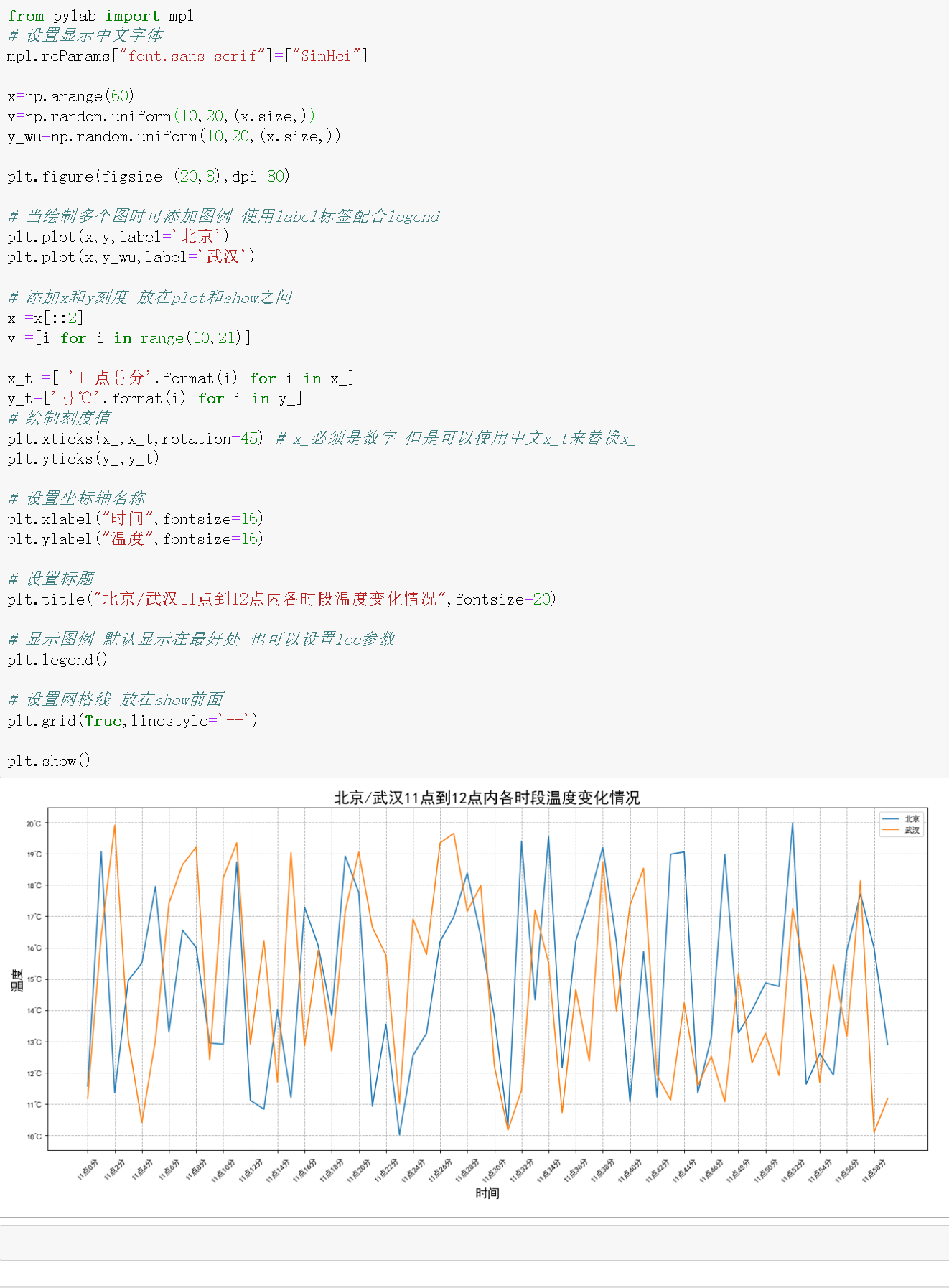## 入门小测试

结合上述案例，想必对于基础绘制过程和函数有一定的了解，现在来做一个小测试吧！仿照上述案例（尤其是案例三）好好想一想奥！

import matplotlib.pyplot as plt
import numpy as np

from pylab import mpl
# 设置显示中文字体
mpl.rcParams["font.sans-serif"]=["SimHei"]

# 准备数据 此处是用于绘制的数据
# 此处要指明数量 否则默认是50 默认是闭区间的
x=np.linspace(1,12,12)
y_2013=np.random.uniform(0,250,(x.size,))
y_2014=np.random.uniform(0,250,(x.size,))
y_2015=np.random.uniform(0,250,(x.size,))

# 创建画布
plt.figure(figsize=(10,10),dpi=100)

# 绘制折线图
plt.plot(x,y_2013,label='2013年')
plt.plot(x,y_2014,label='2014年')
plt.plot(x,y_2015,label='2015年')

# 设置相关参数

# 设置刻度 此处是用于描述刻度的数据
# arange默认是左闭右开区间
x_=[i for i in np.arange(1,13,1)]
y_=[i for i in np.arange(0,250,35)]

x_t=['{}月'.format(i) for i in x_]
y_t=['{}ug/m3'.format(i) for i in y_]

plt.xticks(x_,x_t)
plt.yticks(y_,y_t)

# 设置坐标轴
plt.xlabel("时间",fontsize=16)
plt.ylabel("pm值",fontsize=16)

# 设置标题
plt.title("北京2013年、2014年、2015年pm值",fontsize=20)

# 显示图例
plt.legend()

# 绘制格子
plt.grid(True,linestyle='--')

# 显示图像
plt.show()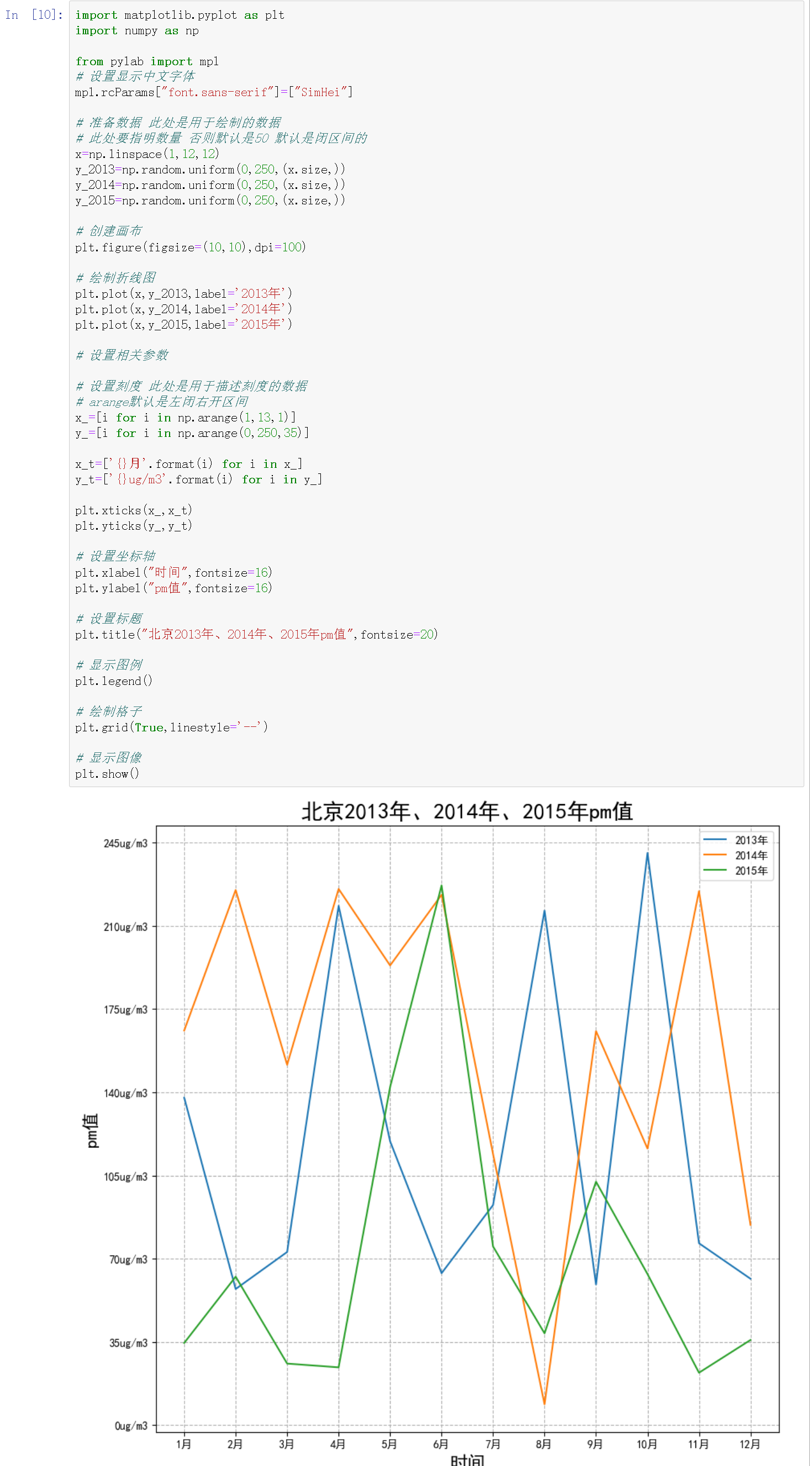更多学习请参考官网！

展开全文python pytorch
• 添加字体到matplot的字体里，然后修改设置。 1、添加字体 可以从网上下载字体，也可以在电脑的Windows的Fonts文件夹里找到字体来使用，这里使用的是Windows里自带“黑体”字体，如下图，然后复制字体文件，添加到...

今天使用Anaconda的matplotlib画图出现了中文乱码，绘出的图片如下：## 解决办法

添加中文字体到matplotlib的字体里，然后修改设置。

1、添加字体
可以从网上下载字体，也可以在电脑的Windows的Fonts文件夹里找到字体来使用，这里使用的是Windows里自带“黑体”字体，如下图，然后复制字体文件，添加到Anaconda下的Lib\site-packages\matplotlib\mpl-data\fonts\ttf 文件夹。2、修改字体设置
在Anaconda下的Lib\site-packages\matplotlib\mpl-data下找到matplotlibrc文件，路径如下：去掉font.family前面的注释，然后把后面的名称改为刚添加的字体名称:simhei，修改字体设置如下，再保存。3、查看结果
关闭Anaconda再重新打开，重新运行，中文乱码就解决好了。展开全文python
• 下载中文字体： ...amp;amp;part=1237887120 将下载的字体ttf文件放到matplotlib字体库位置： anaconda3/lib/python3.6/site-packages/matplotlib/mpl-data/fonts/ttf/ 删除matplotlib字体缓存，文件...font
• 本篇主要针对在MAC系统中Anaconda环境下，matplotlib显示不了中文的问题，提出解决Python绘图时中文显示的方法。 2. 环境 本测试于本人MAC Air机器2018年8月实验成功。 Anaconda： 1.8.7 MacOS： 10.13.6 ...
• 查找Matplotlib默认字体目录 打开anaconda prompt,输入python，接下来进入查找过程 查找代码如下 import matplotlib print(matplotlib.matplotlib_fname()) 结果如下： 红线标注的路径就是Matplotlib的配置文件...python matplotlib
• MAC Python下matplotlib字体配置环境方法一方法二 环境 Mac OS X Catalina 10.15 Python3.7 anaconda 方法一 网上各种通过下载simhei字体，配置matplotlib/matplotlibrc文件夹的方法过于繁琐，且年代久远，Mac OS X...python opencv
• 字体放到matplotlib文件夹中D:\Anaconda3\Lib\site-packages\matplotlib\mpl-data\fonts\ttf 中 修改D:\Anaconda3\Lib\site-packages\matplotlib\font_manager.py文件，保存 修改D:\Anaconda3\Lib\site-packages\...python matplotlib
• E:\software\anaconda3\Lib\site-packages\matplotlib\mpl-data\matplotlibrc 使用文本编辑器打开该文件, 找到 font.sans-serif 所在行，删掉最前面的注释符号,在后面支持的字体添加需要的字体即可， 一般使用黑体...matplotlib SimHei
• mac Anaconda matplotlib 中文乱码问题 GIthub地址，本文中用到的资源下载地址 这里所有的操作都是以黑体字体为例 一....二.... ~/.matplotlib/ ...三. 添加字体matplotlib... 添加字体文件 matplotlib的默认安装目录在
• 用jupyter画图，发现默认字体无法显示，如下图 报错显示是：大概意思是缺少字体 RuntimeWarning: Glyph 21508 missing from current font. font.set_text(s, 0, flags=flags) 一般解决方案 我查询了很多办法，...python
• 一文彻底解决 matplotlib 中的字体管理 如果根据本文，在windows, matplotlib, python 中遇到字体的问题仍然不能自己解决，我先投降！！！ 使用 matplotlib 可视化数据时，经常要面对字体设置的问题。在要用到中文时...python
• 默认字体居然不支持中文字体，必须得吐槽一下~闲言少叙，开始正文方法1：在plot中指定prop参数使用matplotlib.font_manager下的FontProperties加载中文字体调用函数时通过prop属性指定中文字体import matplotlib....
• 字体放到/home/liucc/anaconda3/lib/python3.6/site-packages/matplotlib/mpl-data/fonts/ttf目录下 在调用matplotlib前调用代码 plt.rcParams['font.sans-serif']=['SimHei'] #用来正常显示中文标签...matplotlib
• 在Mac上安装Matplotlib不使用Anaconda，直接使用pip安装即可：pip3 install matplotlib向Matplotlib添加中文字体Microsoft YaHei(微软雅黑)Matplotlib不能默认使用中文字体显示字符的原因只是因为其未包含中文字体...
• 如下图所示，当使用matplotlib绘制图片的时候，所有的中文字符无法正常显示。 三、解决方法 1、下载字体ttf文件 链接:https://pan.baidu.com/s/1RLVvbIi_NpAiiycBYQRPCQ 密码:n1sv 2、找到配置文件 使用如下代码...matplotlib mac
• 这里写自定义目录标题一、环境二、anaconda安装三、jupyter notebook安装配置四、matplotlib中文显示五、参考来源 ​ 一、环境 阿里云centerOS 7 二、anaconda安装 下载 版本根据需求自行选择 #下载 wget ...bash python
• 学习https://matplotlib.org/gallery/index.html ...%matplotlib inline import matplotlib.pyplot as plt plt.rcParams['font.sans-serif']=['SimHei'] # 用来正常显示中文标签 plt.rcParams['axes.unicode_mi...python绘图
• matplotlib 库设置中文显示时会出现小方框，为了解决这个问题查阅了很多资料，但是都是直接在系统环境中修改，而我用的是 Anaconda 自建的环境，无法解决问题，因此查了很久之后找到了解决方法。...添加字体 首先...python
• python matplotlib 在标签、图例显示中文，字体的安装与设置python
• matplotlib给子图添加图例的方法代码如下：import matplotlib.pyplot as pltx = [1,2,3,4,5,6,7,8]y = [5,2,4,2,1,4,5,2]axe1 = plt.subplot(211)s1 = axe1.scatter(x,y, color='r', s=25, marker="o")plt.legend(...
• 1.下载中文arial unicode ms字体到你系统的/home 目录或其他目录（只要你找得到） 2. 拷贝字体到 usr/share/fonts 下： 2.1查看字体库目录 一般在 /usr/share/fonts/文件夹内 fc-list 2.2 首先把下载的字体...matplotlib python
• 原因：matplotlib找不到字体，需要自行设置 解决方案一： Linux系统： windows系统： 第一步：将中文字体复制到matplotlib字体目录中 中文字体以微软雅黑为例，在 C:\Windows\Fonts 下面找到“微软雅黑”字体。...python
• matplotlib调用中文字体，运行plt.rcParams['font.sans-serif'] = ['SimHei']报错解决办法python anaconda pycharm
• 今天上午画小论文图的时候，用matplotlib画，发现如果设置中文的标题会出现方块的问题，在网上看了许多教程，可以总结为以下几个：① 每次编写代码时进行参数设置# coding: UTF-8 import matplotlib.pyplot as plt ...utf-8...